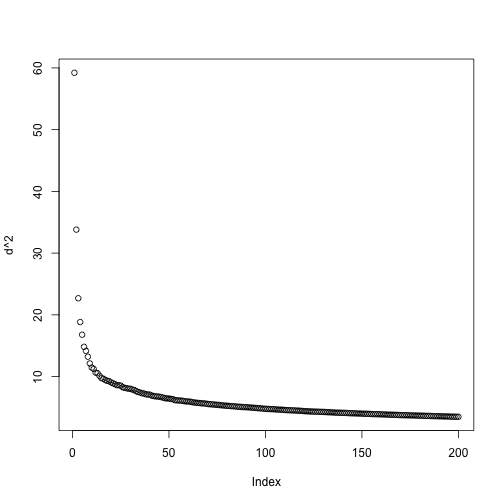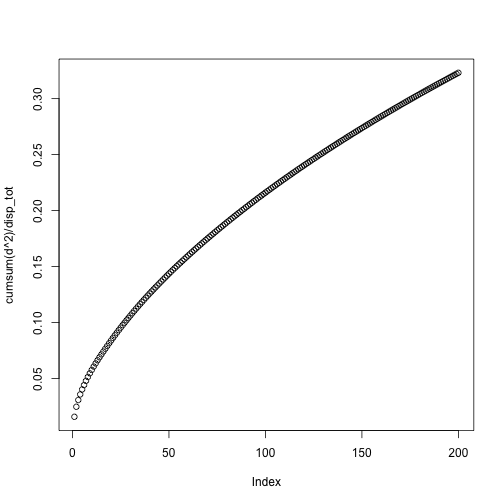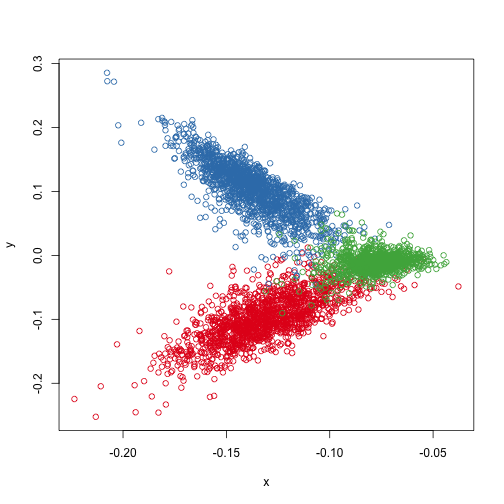# Matrix Decompositions

Patrick O. Perry, NYU Stern School of Business

## Preliminaries

### Computing environment

We will use the following R packages.

``````library("jsonlite")
library("Matrix")
library("rARPACK")
library("tm")
``````

To ensure consistent runs, we set the seed before performing any analysis.

``````set.seed(0)
``````

### Data

We will analyze the SMART Classic3 Corpus. This is a standard corpus for evaluating document clustering methods. Unfortunately, the original version appears to be unavailable. I was able to find a version of the data [hosted by Volkan Tunaliy(http://www.dataminingresearch.com/index.php/2010/09/classic3-classic4-datasets/). I have combined Tunali's version into a single JSON file, which we can read directly.

``````classic <- jsonlite::stream_in(file("classic3.json"), verbose=FALSE)
``````

The corpus contains abstracts from three different collections

``````table(classic\$collection)
``````
``````
CISI CRAN  MED
1460 1398 1033
``````

The descriptions of the collections are as follow

• CISI: library science
• CRAN: Cranfield collection; publications from aeronautic reviews
• MED: Medlars collection; publications from medical reviews

## Document-Term Matrix

``````corpus <- VCorpus(VectorSource(classic\$text))
control <- list(tolower = TRUE, removePunctuation = TRUE,
removeNumbers = TRUE, stemming = TRUE,
stopwords = TRUE, weighting=weightTfIdf)
dtm <- DocumentTermMatrix(corpus, control)
dtm <- sparseMatrix(dtm\$i, dtm\$j, x = dtm\$v, dim=dim(dtm),
dimnames=dimnames(dtm))
``````

## Decompositions

With a large matrix, we usually cannot afford to compute the entire SVD. Instead, we compute the top 200 singular values and vectors using the `svds` function, from the `rARPACK` package.

``````dtm_svd <- svds(dtm, 200)
``````

For principal components analysis, we would usually “center” the matrix before computing the singular value decomposition. That is, we would subtract the column means from each row of the matrix. Then, the right singular vectors would be the eigenvectors of the sample covariance matrix. The problem with doing so is that centering the matrix makes it dense. So, in practice, most people avoid the centering step. That means that instead of talking about variance below, I talk about “dispersion” below, I mean dispersion around zero (variance is dispersion around the mean).

To see how much dispersion is explained by each component, look at the squares of the singular values:

``````d <- dtm_svd\$d
plot(d^2)
``````The total dispersion is equal to the sum of squares of all singular values, which is equal to the sum of squares of all elements of the data matrix.

``````(disp_tot <- sum(dtm^2))
``````
`````` 3769.374
``````

The following graph shows the cumulative dispersion explained by the leading components of the singular value decomposition:

``````plot(cumsum(d^2) / disp_tot)
``````We can see that with 200 components, we are explaining over 30% of the variability in the data. The original data matrix has dimensions

``````dim(dtm)
``````
``````  3891 15736
``````

so this is a substantial reduction.

``````terms <- colnames(dtm)

for (i in 1:5) {
cat("Top 10 terms for vector ", i, ":\n", sep="")
ix <- rank(-abs(dtm_svd\$v[,i])) <= 10
print(data.frame(term=terms[ix], weight=round(dtm_svd\$v[ix,i], 2)))
cat("\n")
}
``````
``````Top 10 terms for vector 1:
term weight
1  boundari  -0.09
2      flow  -0.14
3    inform  -0.15
4   librari  -0.16
5    method  -0.10
6    number  -0.10
7   pressur  -0.10
8   problem  -0.09
9    system  -0.12
10      use  -0.09

Top 10 terms for vector 2:
term weight
1  boundari   0.13
2    cylind   0.11
3      flow   0.20
4     index  -0.11
5    inform  -0.21
6     layer   0.14
7   librari  -0.26
8     plate   0.12
9   pressur   0.14
10   system  -0.15

Top 10 terms for vector 3:
term weight
1     acid  -0.10
2     cell  -0.35
3   growth  -0.13
4   hormon  -0.13
5   inform   0.12
6  librari   0.12
7  patient  -0.22
8      rat  -0.16
9    tissu  -0.11
10   tumor  -0.10

Top 10 terms for vector 4:
term weight
1    axial  -0.14
2     bend  -0.14
3    buckl  -0.39
4    creep  -0.14
5   cylind  -0.26
6  cylindr  -0.15
7     flow   0.15
9    shell  -0.37
10  stress  -0.21

Top 10 terms for vector 5:
term weight
1   automat  -0.13
2      book   0.13
3   catalog   0.16
4  document  -0.22
5     index  -0.27
6    inform  -0.21
7   librari   0.59
8   retriev  -0.19
9    search  -0.14
10   system  -0.14
``````

## Document Visualization

Here are the scores for the first two factors for all 3891 abstracts, colored by collection.

``````x <- dtm_svd\$u[,1] * dtm_svd\$d
y <- dtm_svd\$u[,2] * dtm_svd\$d
plot(x, y, col=as.integer(factor(classic\$collection)))
``````## Clustering

We can cluster the documents by applying k-means to the left singular vectors, scaled by their relative importances (the corresponding singular values):

``````cl <- kmeans(dtm_svd\$u %*% diag(dtm_svd\$d), 3, nstart=100)
``````

The output of k-means is highly dependent on the random initialization. To get reliable results from k-means, I use a large number of random initializations.

We can see that the clustering was able to recover the original document classifications:

``````table(classic\$collection, cl\$cluster)
``````
``````
1    2    3
CISI    4    0 1456
CRAN    2 1377   19
MED  1015    2   16
``````

If we don't do any sort of dimensionality reduction, the results are similar in this application, but, it takes much longer.

``````# Not run, because it takes too long:
##dtm_dense <- as.matrix(dtm) # k-means needs a dense matrix
##cl0 <- kmeans(dtm_dense, 3, nstart=100)
``````

## Session information

``````sessionInfo()
``````
``````R version 3.2.3 (2015-12-10)
Platform: x86_64-apple-darwin13.4.0 (64-bit)
Running under: OS X 10.10.5 (Yosemite)

locale:
 en_US.UTF-8/en_US.UTF-8/en_US.UTF-8/C/en_US.UTF-8/en_US.UTF-8

attached base packages:
 methods   stats     graphics  grDevices utils     datasets  base

other attached packages:
 tm_0.6-2           NLP_0.1-8          rARPACK_0.10-0     Matrix_1.2-3       jsonlite_0.9.16
 RColorBrewer_1.1-2 knitr_1.12.3

loaded via a namespace (and not attached):
 Rcpp_0.11.5      codetools_0.2-14 lattice_0.20-33  digest_0.6.8     slam_0.1-32
 plyr_1.8.1       grid_3.2.3       formatR_1.1      magrittr_1.5     evaluate_0.8
 stringi_1.0-1    tools_3.2.3      stringr_1.0.0    parallel_3.2.3
``````